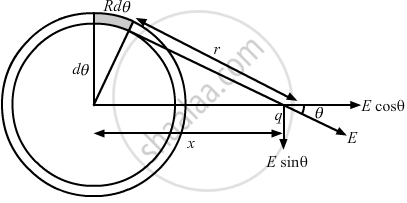Advertisement Remove all ads

# A Positive Charge Q is Distributed Uniformly Over a Circular Ring of Radius R. a Particle of Mass M, and a Negative Charge Q, is Placed on Its Axis at a Distance X from the Centre. - Physics

Short Note

A positive charge Q is distributed uniformly over a circular ring of radius R. A particle of mass m, and a negative charge q, is placed on its axis at a  distance x from the centre. Find the force on the particle. Assuming x << R, find the time period of oscillation of the particle if it is released from there .

Advertisement Remove all ads

#### Solution

Consider an element of angular width $d\theta$  at a distance r from the charge q on the circular ring, as shown in the figure.So, charge on the element,
$dq = \frac{Q}{2\pi R}Rd\theta = \frac{Q}{2\pi}d\theta$
Electric field due to this charged element,

$dE = \frac{dq}{4\pi \epsilon_0}\frac{1}{r^2}$

$= \frac{\frac{Q}{2\pi}d\theta}{4\pi \epsilon_0}\frac{1}{R^2 + x^2}$

By the symmetry, the E sinθ component of all such elements on the ring will vanish.
So, net electric field,

$d E_{net} = dE\cos\theta = \frac{Qd\theta}{8 \pi^2 \epsilon_0 \left( R^2 + x^2 \right)^{3/2}}$

Total force on the charged particle,

$F = \int qd E_{net}$

$= \frac{qQ}{8 \pi^2 \epsilon_0}\frac{x}{\left( R^2 + x^2 \right)^{3/2}} \int_0^{2\pi} d\theta$

$= \frac{xQq}{4\pi \epsilon_0 \left( R^2 + x^2 \right)^{3/2}}$

According to the question,

$x < < R$

$F = \frac{Qq x}{4\pi \epsilon_0 R^3}$

Comparing this with the condition of simple harmonic motion, we get

$F = m \omega^2 x$

$\Rightarrow m \omega^2 = \frac{Qq}{4\pi \epsilon_0 R^3}$

$\Rightarrow m \left( \frac{2\pi}{T} \right)^2 = \frac{Qq}{4\pi \epsilon_0 R^3}$

$\Rightarrow T = \left[ \frac{16 \pi^3 \epsilon_0 m R^3}{Qq} \right]^{1/2}$

Concept: Electric Charges
Is there an error in this question or solution?
Advertisement Remove all ads

#### APPEARS IN

HC Verma Class 11, Class 12 Concepts of Physics Vol. 2
Chapter 7 Electric Field and Potential
Q 39 | Page 122
Advertisement Remove all ads

#### Video TutorialsVIEW ALL 

Advertisement Remove all ads
Share
Notifications

View all notifications

Forgot password?